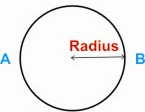HOME MATH DICTIONARY DOWNLOAD FEEDBACK DISCLAIMER
 Question: What is Radius ? Answer: A radius of a circle is any straight line drawn from the center of the circle to a point on its circumference. The phrase the radius of a circle is often taken to mean the length of the radius of the circle. So for example, if asked to find the radius of a bicycle wheel it is the length of its radius which is required. The radius of a circle is the distance from the centre of the circle to its edge. The radius of a regular polygon is the distance from the centre of the polygon to any one of its vertices. The plural of radius is radii.Example of Radius of the Circle: